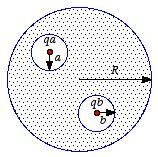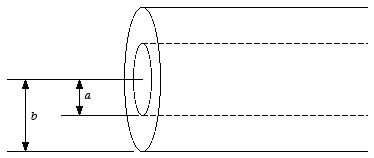# Home Work Set # 5, Physics 217, Due: October 10, 2001

## Problem 1

Two spherical cavities, of radii a and b, are hollowed from the interior of a neutral conducting sphere of radius R (see Figure 1). At the center of each cavity a point charge is placed; call these charges qa and qb.Figure 1. Problem 1.
a) Find the surface charge densities σa, σb and σR.
b) What is the field outside the conductor?
c) What is the field within each cavity?
d) What is the force on qa and qb?
e) Which of these answers would change if a third charge, qc, were brought near the conductor?

## Problem 2

A metal sphere of radius R carries a total charge Q. What is the force of repulsion between the "northern" and the "southern" hemisphere.

## Problem 3

Find the capacitance per unit length of two coaxial metal cylindrical tubes, of radii a and b (see Figure 2).Figure 2. Problem 3.